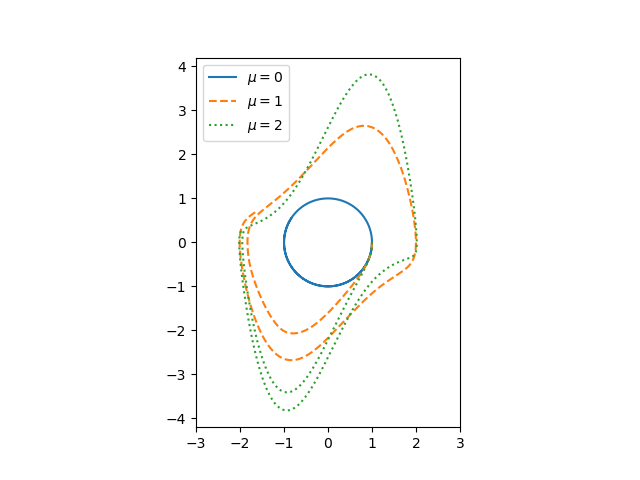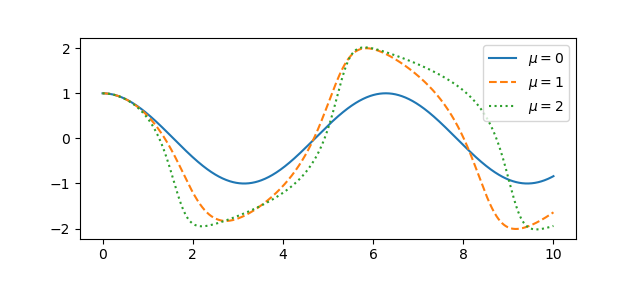# Solving Van der Pol equation with ivp_solve

Van der Pol’s differential equation isThe equation describes a system with nonlinear damping, the degree of nonlinearity given by μ. If μ = 0 the system is linear and undamped, but as μ increases the strength of the nonlinearity increases. We will plot the phase portrait for the solution to Van der Pol’s equation in Python using SciPy’s new ODE solver ivp_solve.

The function ivp_solve does not solve second-order systems of equations directly. It solves systems of first-order equations, but a second-order differential equation can be recast as a pair of first-order equations by introducing the first derivative as a new variable.Since y is the derivative of x, the phase portrait is just the plot of (x, y).If μ = 0, we have a simple harmonic oscillator and the phase portrait is simply a circle. For larger values of μ the solutions enter limiting cycles, but the cycles are more complicated than just circles. These limiting cycles are periodic attractors: every non-trivial solution converges to the limit cycle.

Here’s the Python code that made the plot.

from scipy import linspace
from scipy.integrate import solve_ivp
import matplotlib.pyplot as plt

def vdp(t, z):
x, y = z
return [y, mu*(1 - x**2)*y - x]

a, b = 0, 10

mus = [0, 1, 2]
styles = ["-", "--", ":"]
t = linspace(a, b, 500)

for mu, style in zip(mus, styles):
sol = solve_ivp(vdp, [a, b], [1, 0], t_eval=t)
plt.plot(sol.y, sol.y, style)

# make a little extra horizontal room for legend
plt.xlim([-3,3])
plt.legend([f"$\mu={m}$" for m in mus])
plt.axes().set_aspect(1)


To plot the solutions as a function of time, rather than plotting phase portraits, change the line

    plt.plot(sol.y, sol.y, style)

to

    plt.plot(sol.t, sol.y, style)

and comment out the line setting xlim This gives the following plot.## One thought on “Solving Van der Pol equation with ivp_solve”

1. Charlie Harrison

I think you would be amazed at the applications that rely on the assessment of the damping characteristics of a system. Although I am a simply geotechnical engineer who focuses on soil and rock, we still study the damping and amplification of natural materials affected by earthquake and blast induced vibrations, and so many others. The ralated post on mechanical vibrations that is referenced is more familar to me, as the same equations are used to design foundations to support oscillating equipment, such as a ball mill in a ore processing plant at a mine or a generator in a hydroelectric powerhouse. Absolutely fascinating stuff!!!

Thanks for all the great posts in 2019! Have a great holiday season!

Can’t wait to see what you have to share in 2020!!!